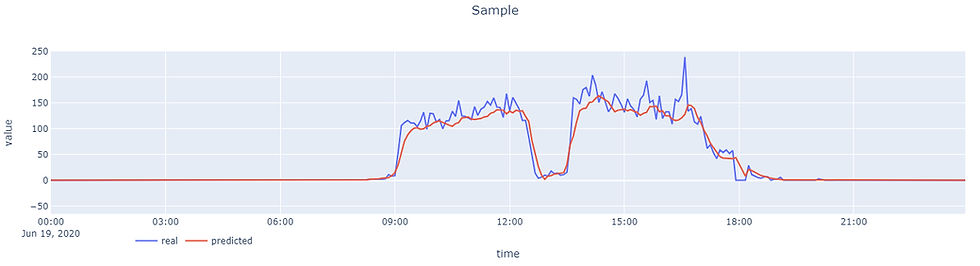top of page
Search
• Oktay Sahinoglu

# Anomaly (Outlier) Detection with Unlabeled Time Series Data

Updated: Oct 5, 2020In real life, labeling data is not always easy or possible. For example, think about thousands of sensors providing data every minute, so it is not easily possible to label every single sample of these thousands signals for every minute. However, the need for anomaly detection is there and we need to find a solution for that.You may assume that the model, mentioned in this post, is an LSTM model for predictive maintenance with time series data, which has a pattern, received from sensors or systems. Proposed features and predictions also belong to this model. You can find the model details here. However, the focus of this post is the holistic concepts which also include how we will use this prediction.

First of all, let's take a sample time series signal received from a sensor to work with. Below you can see one day graph of an sample signal.Labeled DataWith labeled data as shown, we can consider anomaly detection as a classification problem. We know all the anomaly points beforehand and we can set up and train our Machine Learning model to predict anomaly label as 1 or 0, which is a classification. Our features would be parts of time, a distinctive identity of the signal if we have multiple signals, and value of the signal. Output would be the anomaly label.

Unlabeled DataOn the other hand, if we have an unlabeled data, which is the case of this post, we only have time steps and the value of our signal as shown. Now, we have to change our approach to the problem. We will be trying to predict the value itself instead of a label. The problem turns into regression instead of classification. There may be different kind of approaches for forecasting the time series. Statistical methods such as ARIMA can be used as well as Machine Learning based models such as LSTM or CNN for example. Our features will be again parts of time and a distinctive identity of the signal if we have multiple signals. Value of the signal for previous time-steps can be used in a different way which will be mentioned in model details post. However, this time the output will be the value of the signal.

• You can find the details about time features and how to encode them here.

• Distinctive identity of the signal can be thought as one-hot encoded feature. But, if you have hundreds or thousands of signals, then one-hot encoded vector will be a very high dimensional vector. To reduce the dimension of a vector, we can use Embedding. You can find detailed information about Embedding here.

• Model details are mentioned here.

Ok. We succeeded in prediction for the value of the signal.Prediction shown above was produced by an LSTM model.

Our model is supposed to learn the time basis pattern of the signal and make prediction.

Anomaly decision

How do we know whether there is an anomaly or not?

There is always a difference between the predicted value and the real value, which is generally more fluctuated and may contain some noise, produced by a sensor or a system. This difference is the key point to detect the anomaly. The greater the difference, the more likely it is to be abnormal.

How big a difference we need to raise the flag of anomaly?

As mentioned above, the real value is generally more fluctuated and may contain some noise. The question then becomes how tolerant you want to be towards these fluctuations. Based on this decision, you should set the upper and lower bounds of confidence interval. The details of setting the upper and lower bounds of confidence interval for a signal are here. When you apply the confidence interval over your predicted value, you will have...We can now raise the anomaly flag where the real value falls outside the confidence interval.Is it enough just to raise the flag?

If you don't deal with the amount of abnormality, yes it is enough. However, are the criticalities of the anomaly on the left and anomaly on the right marked in the graphic above same? If you would like to see how worse the anomaly is, then we should do one more thing.

Anomaly score

To demonstrate better, I modified the real value data a bit.We can use this ratio as starting point. Because it gives a value in;

[0, 1] → [0,1] * 100 = [0, 100] → Anomaly Score!!

However, for some signals you may get mostly zeros and "a" and "b" values may end up with zero. Then we have problem of division by zero.To overcome the division by zero, we can use arctan of the same ratio. Angle of this ratio will give a value in [0, π/4].

[0, π/4] / (π/4) → [0,1] * 100 = [0, 100]

[0, 100] → Anomaly Score!!

That's all. Hope you like it. See you in another post.